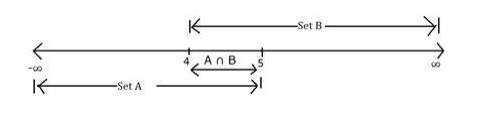# Solve this

Question:

If $A=\{x: x \in R, x<5\}$ and $B=\{x: x \in R, x>4\}$, find $A \cap B$.

Solution:

$A=\{x: x \in R, x<5\}$

As $x$ takes all real values upto 5 hence the set $A$ will contain all numbers from $-\infty$ to 5

$A=(-\infty, 5)$

$B=\{x: x \in R, x>4\}$

As $x$ takes all real values greater than 4 hence the set $B$ will contain values from 4 to $\infty$

$B=(4,-\infty)$

Hence their intersection or the common part between sets $A$ and $B$ would be values from 4 to 5

Hence $A \cap B=(4,5)$

Representing the sets on number line